Courses

# Scalar and Vector Potentials - Electromagnetic Theory, CSIR-NET Physical Sciences Physics Notes | EduRev

## Physics : Scalar and Vector Potentials - Electromagnetic Theory, CSIR-NET Physical Sciences Physics Notes | EduRev

The document Scalar and Vector Potentials - Electromagnetic Theory, CSIR-NET Physical Sciences Physics Notes | EduRev is a part of the Physics Course Physics for IIT JAM, UGC - NET, CSIR NET.
All you need of Physics at this link: Physics

• The electric scalar and magnetic vector potentials.

• The wave equations for the electromagnetic potentials.

Potentials

A potential is a function whose derivative gives a field. Fields are associated with forces; potentials are associated with energy.
The magnetic vector potential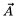is defined so that the magnetic field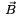is given by: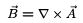(1)

The electric scalar potential φ is defined so that the electric field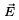is given by: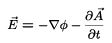( 2 )

Note that in general, the scalar and vector potentials are functions of position and time.

Electrostatic Potential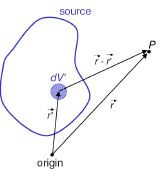The electric field in the presence of a static charge distribution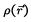is found from Coulomb’s law: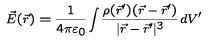(3)

where the integral extends over all space. Note that the prime on the coordinates indicates that the coordinate is associated with the charge.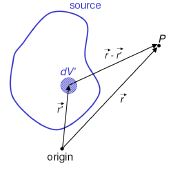In terms of the scalar potential, for a static charge distribution, we have: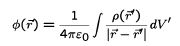(4)

Calculating the potential is simpler than calculating the field directly; and one can then use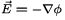to find the electric field.

Since we have from Maxwells’ equations: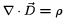(5)

where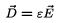(6)

it follows that in an homogeneous, isotropic medium: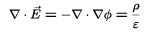(7)

and so: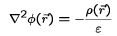(8)

Equation (8) is called Poisson’s equation.

Equation (4) is the solution to Poisson’s equation, expressed as an integral.

The behaviour of a charged particle in an electric field is determined by the field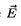, rather than by the potential.

Since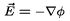for an electrostatic field, we can add any function with vanishing gradient to the potential φ, and obtain the same physics. In other words, the behaviour of any electrostatic system is the same under the transformation: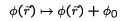(9)

where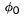is a constant (independent of position).

The freedom that we have in choosing the potential is called gauge invariance.
This allows us to choose arbitrarily the point at which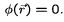Note that if we write the solution to Poisson’s equation (4):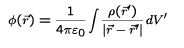(10)

then implicitly (assuming that all charges are within a finite distance from the origin), we make the gauge choice: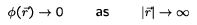(11)

In the presence of sources for the magnetic field (i.e. a current distribution), the magnetic field B can be found from the Biot-Savart law: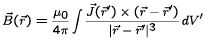( 1 2 )

where the integral extends over all space.
Generally, the Biot-Savart law is difficult to apply.
It is often easier to first calculate the magnetic vector potential; but first, we need to derive the differential equation for the vector potential.

In a static case (constant fields, charges and currents), the magnetic field is related to the current density by: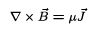(13)

Substituting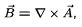, and using the vector identity: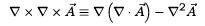(14)

we find: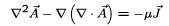(15)

This looks like a complicated equation; but there is a way to simplify it...

Suppose that: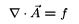(16)

where f is some function of position. Let us define a new vector potential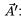: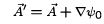(17)

Since: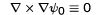(18)

for any function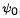the new vector potential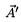gives exactly the same magnetic field as the old vector potential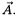.

However, if we choose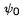such that: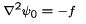( 19)

then: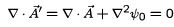(20)

In other words, given a vector potential, we can always choose to work with another vector potential that gives the same field as the original one, but that has zero divergence.

Assuming that we make such a choice, then equation (15) for the vector potential becomes: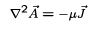(21)

This is again Poisson’s equation - or rather, three Poisson equations, one for each component of the vectors involved.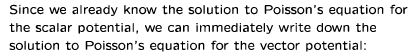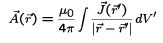( 22 )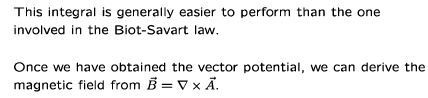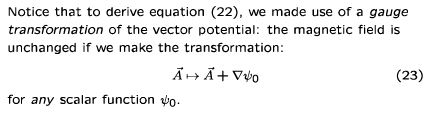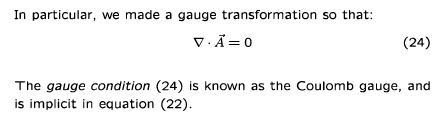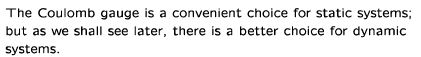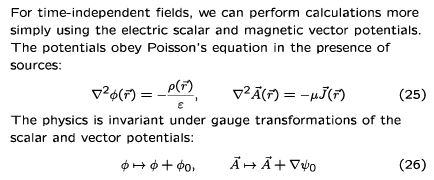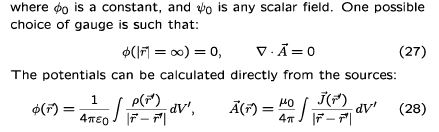Offer running on EduRev: Apply code STAYHOME200 to get INR 200 off on our premium plan EduRev Infinity!

159 docs

,

,

,

,

,

,

,

,

,

,

,

,

,

,

,

,

,

,

,

,

,

,

,

,

;